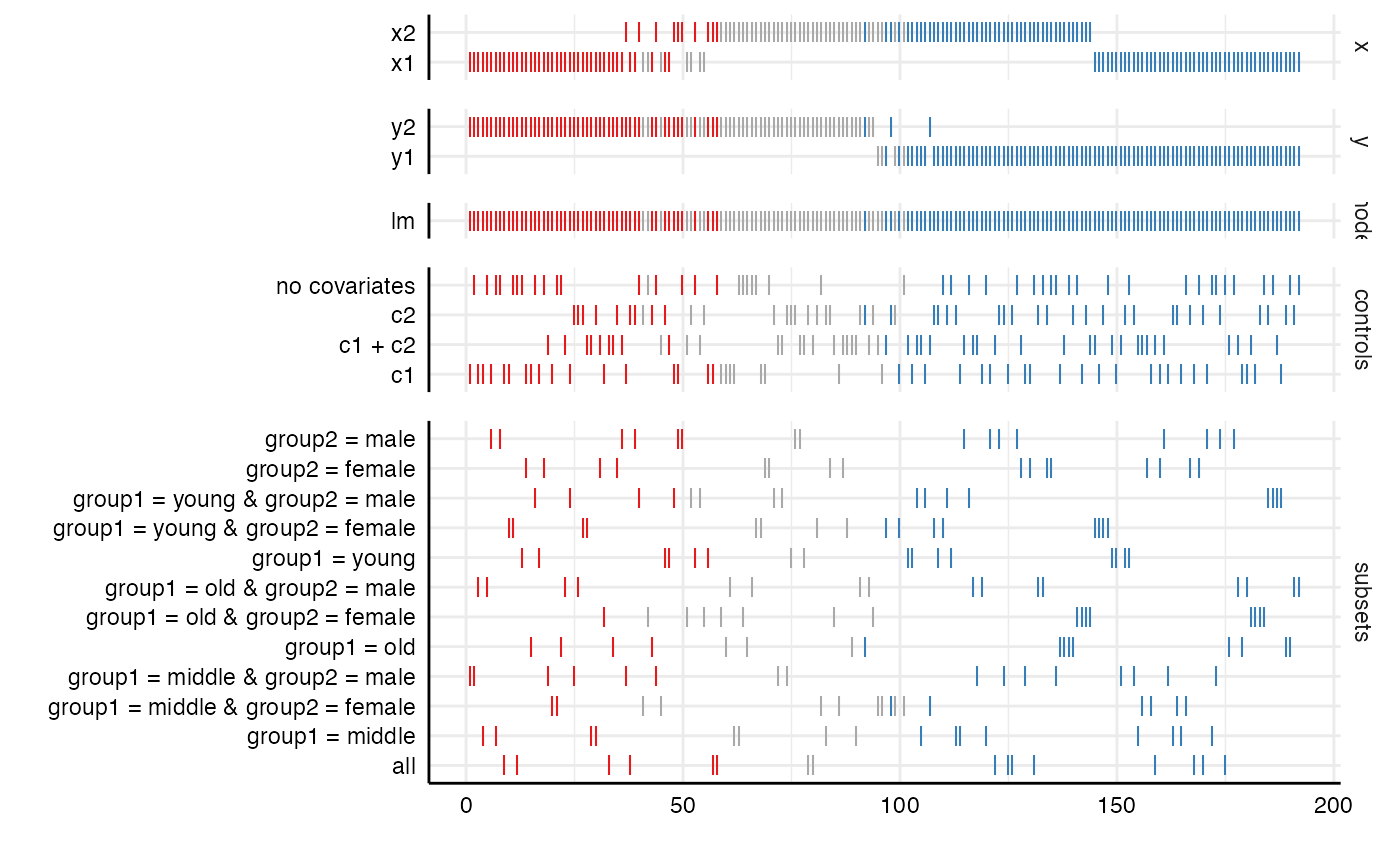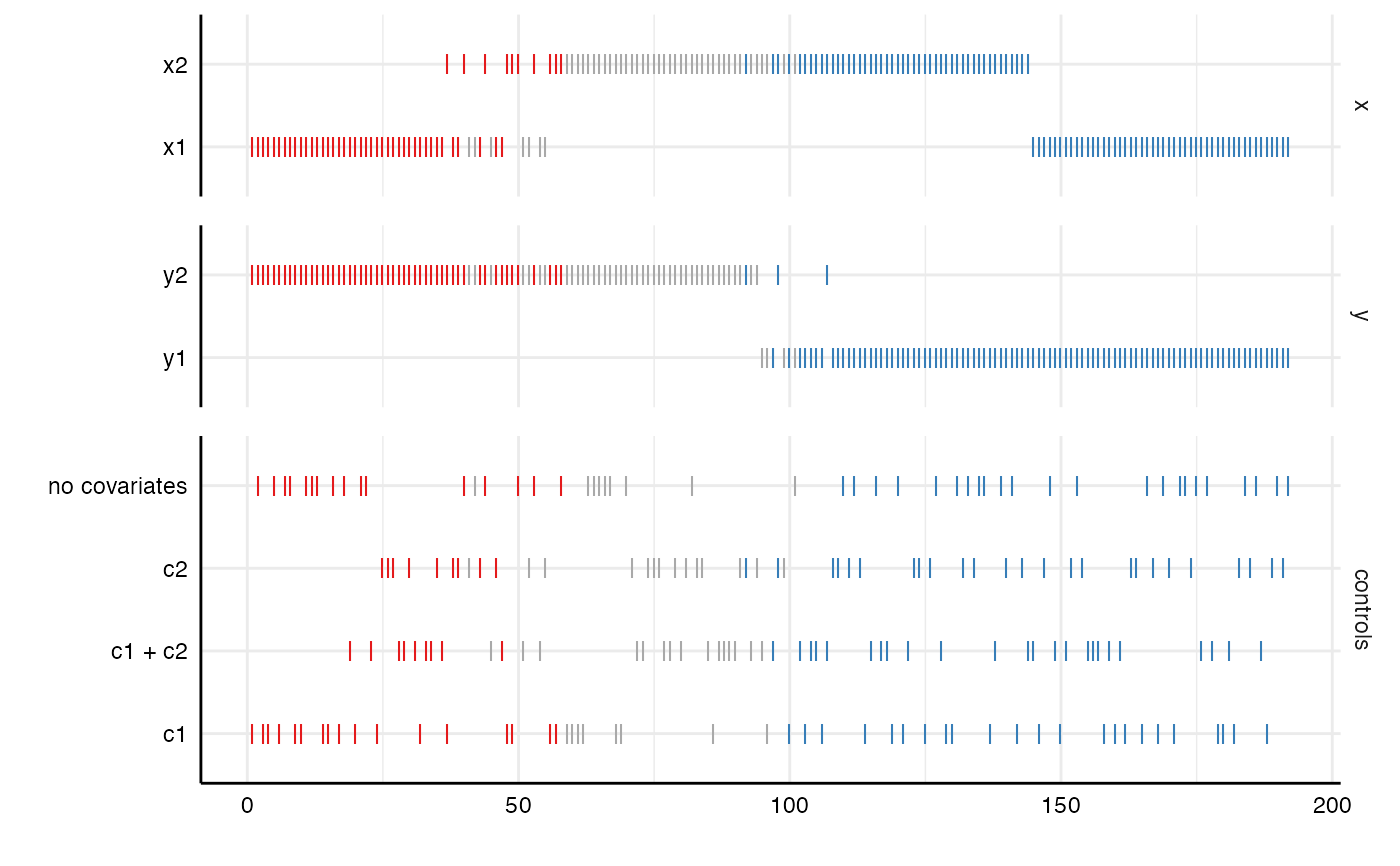This functions plots how analytical choices affect the obtained results (i.e., the rank within the curve). Significant results are highlighted (negative = red, positive = blue, grey = nonsignificant). This functions creates the lower panel in plot_specs().

plot_choices(
df,
var = .data$estimate, choices = c("x", "y", "model", "controls", "subsets"), desc = FALSE, null = 0 ) ## Arguments df a data frame resulting from run_specs(). var which variable should be evaluated? Defaults to estimate (the effect sizes computed by run_specs()). choices a vector specifying which analytical choices should be plotted. By default, all choices are plotted. desc logical value indicating whether the curve should the arranged in a descending order. Defaults to FALSE. null Indicate what value represents the 'null' hypothesis (Defaults to zero). ## Value a ggplot object. ## Examples # Run specification curve analysis results <- run_specs(df = example_data, y = c("y1", "y2"), x = c("x1", "x2"), model = c("lm"), controls = c("c1", "c2"), subsets = list(group1 = unique(example_data$group1),
group2 = unique(example_data\$group2)))

# Plot simple table of choices
plot_choices(results)# Plot only specific choices
plot_choices(results,
choices = c("x", "y", "controls"))